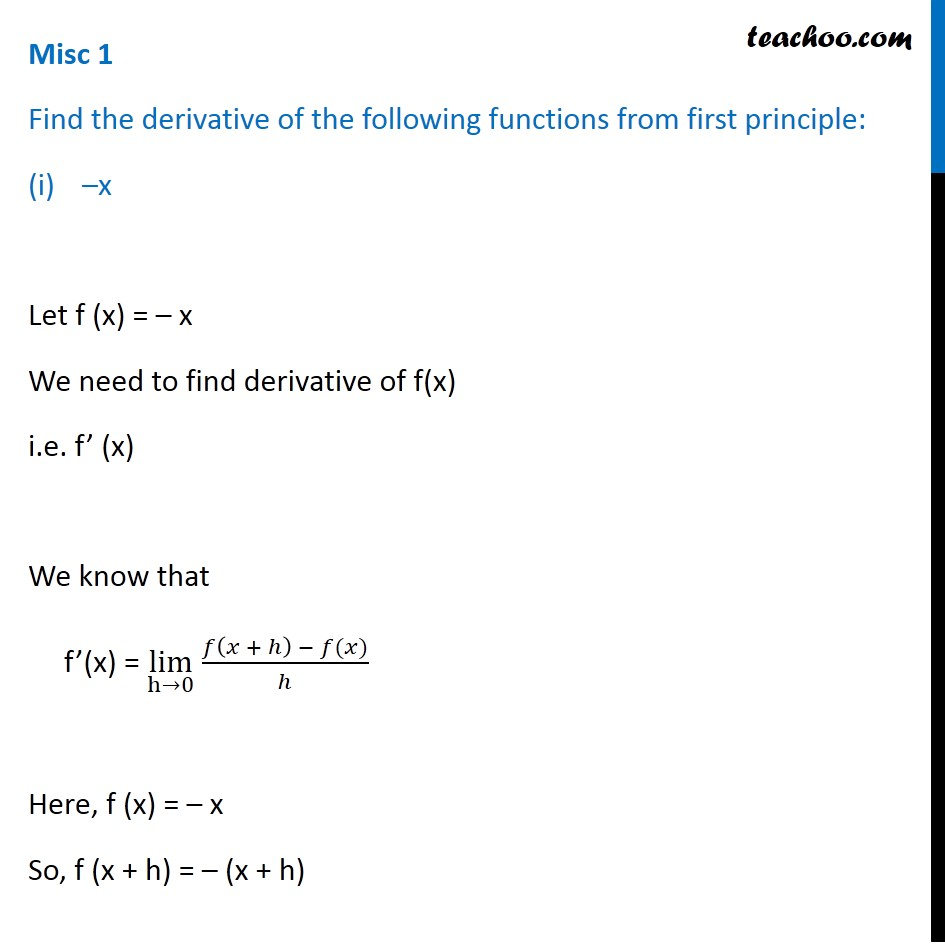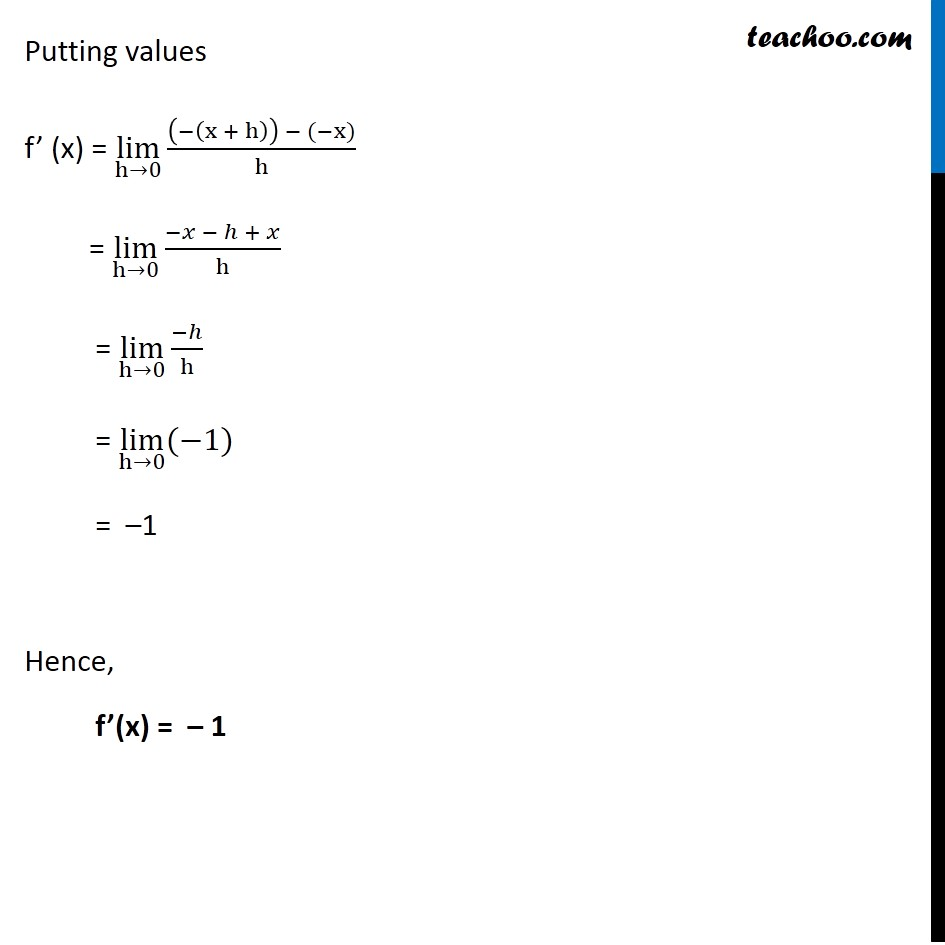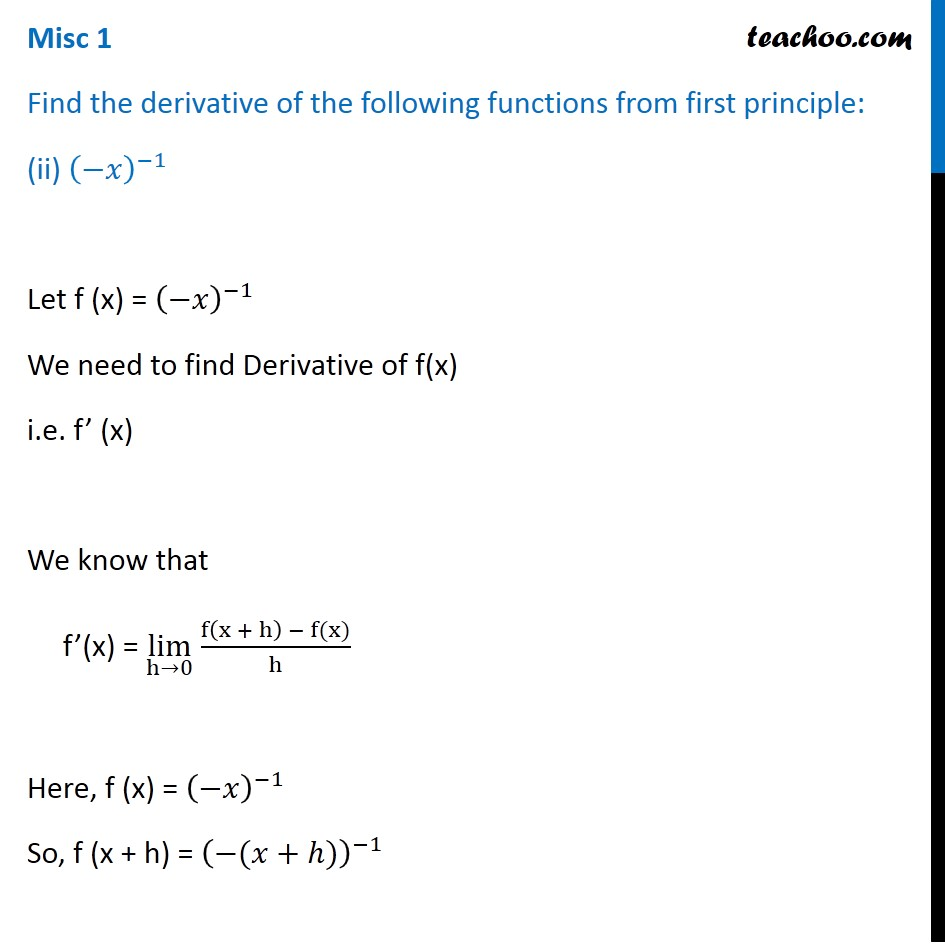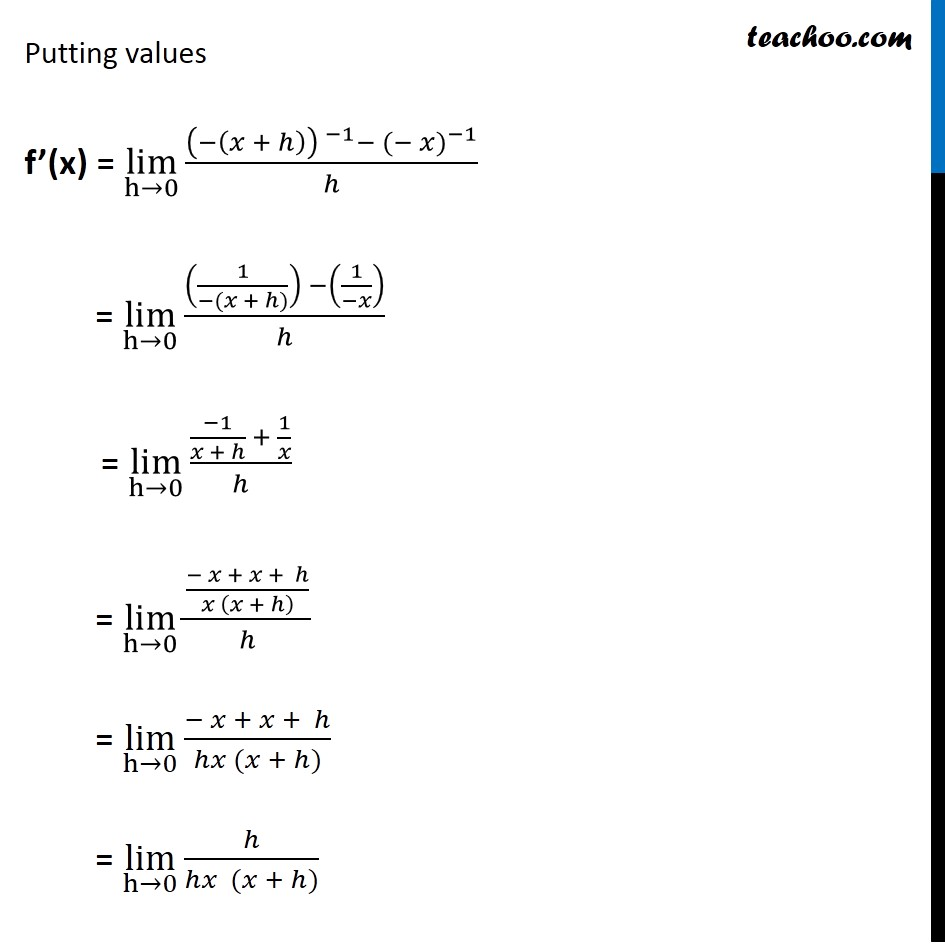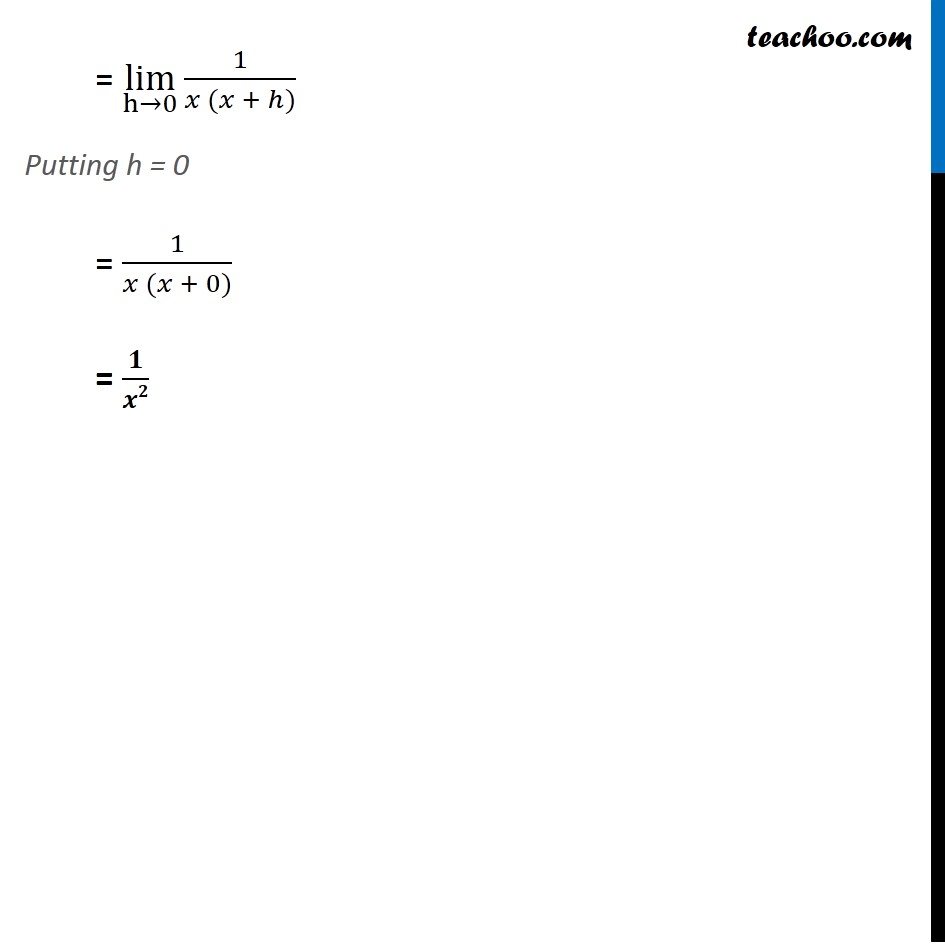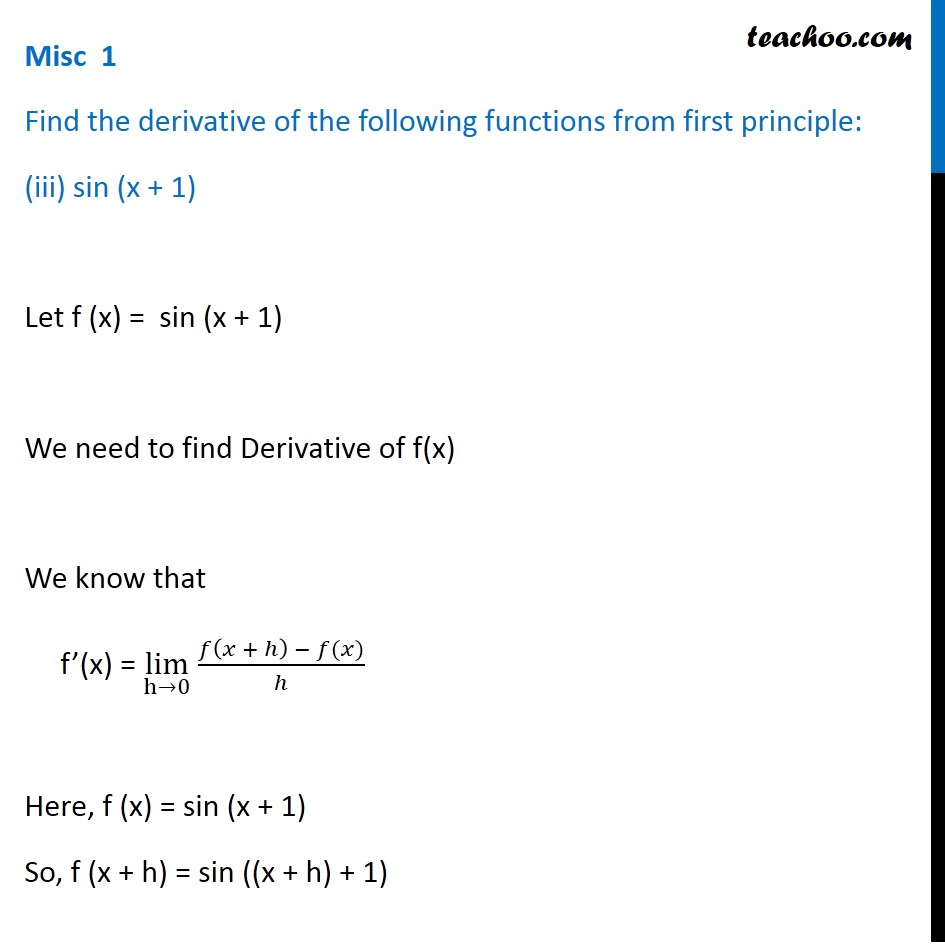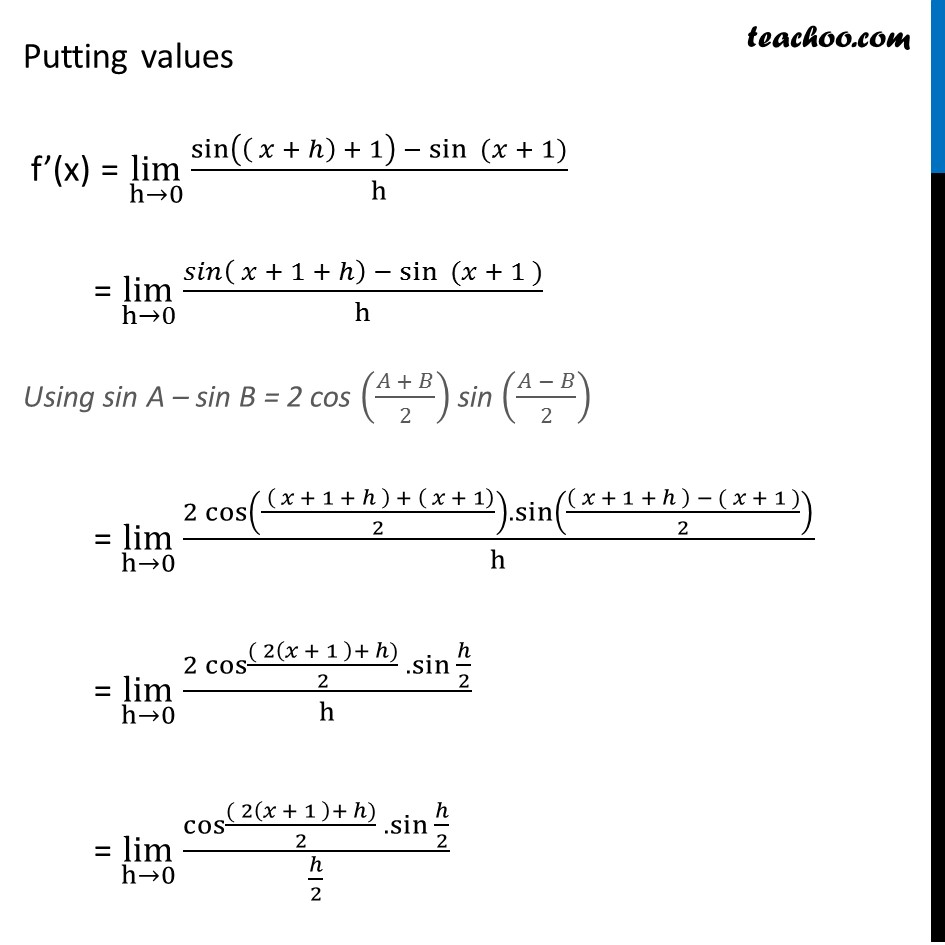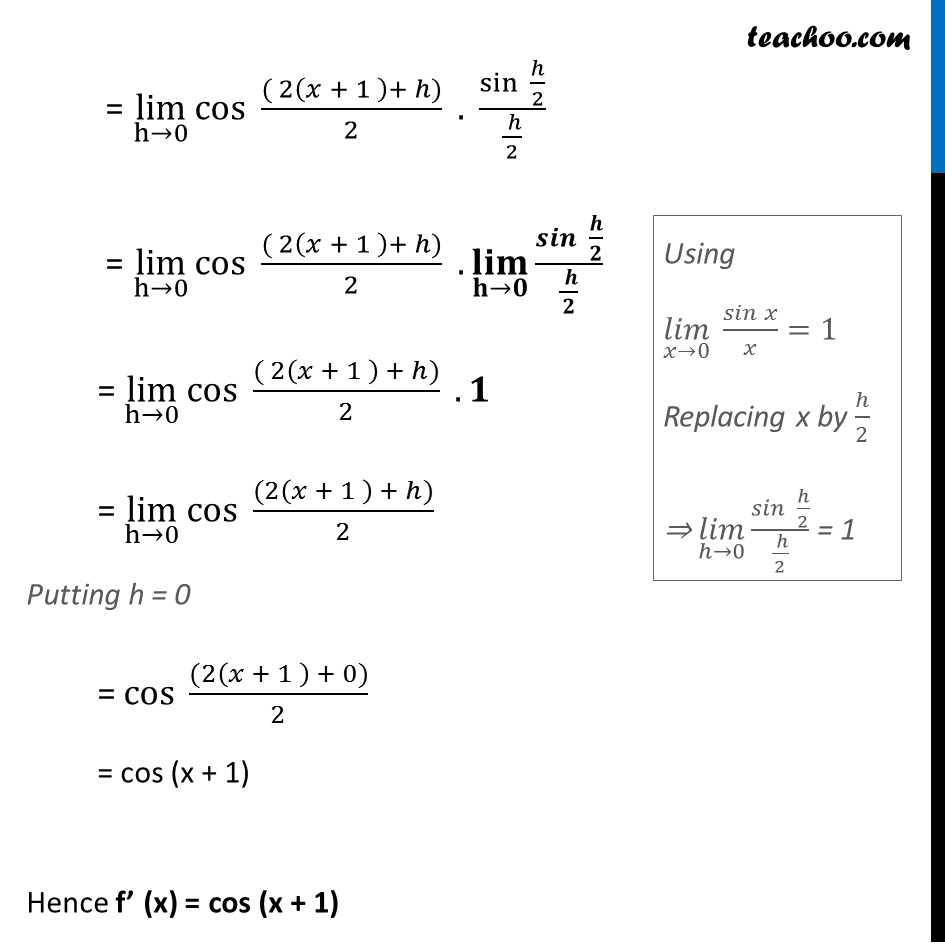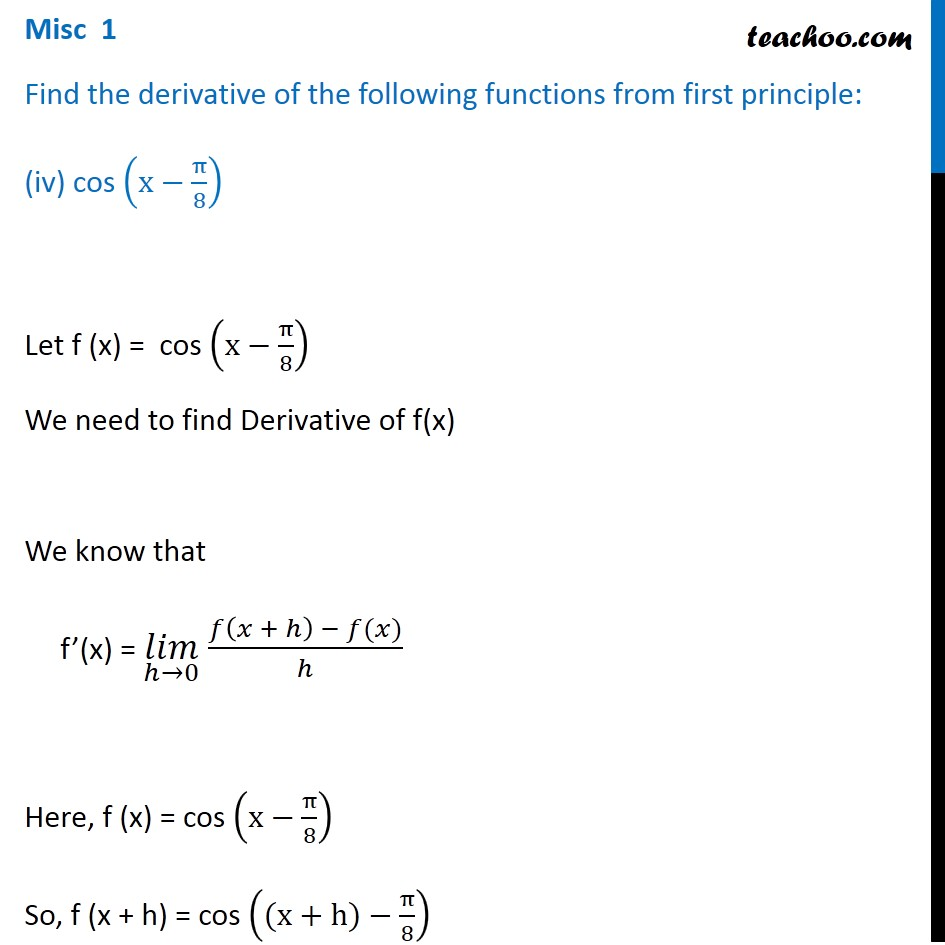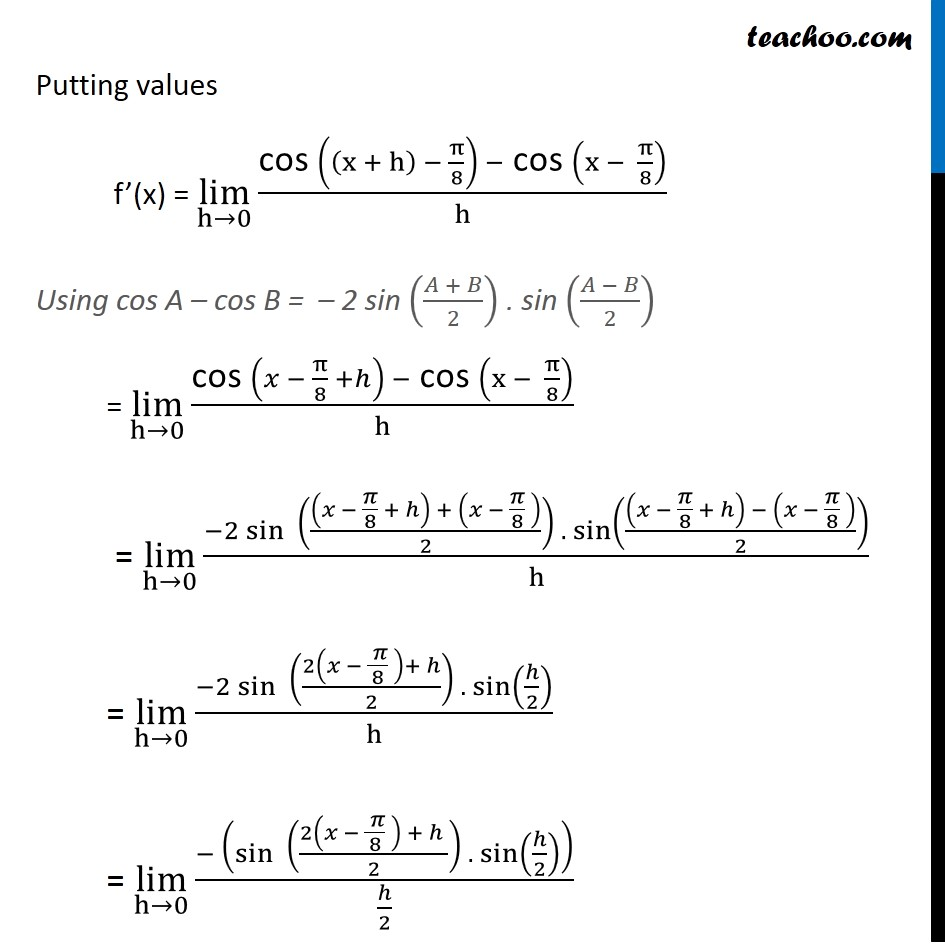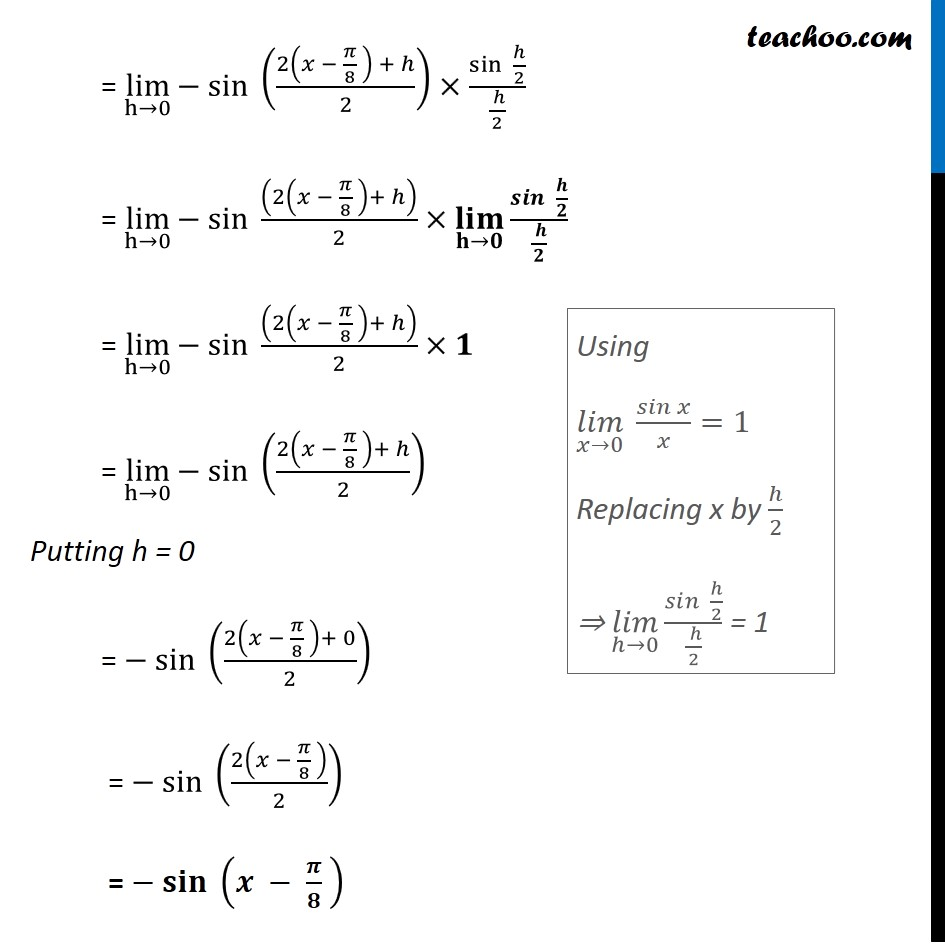Subscribe to our Youtube Channel - https://you.tube/teachoo

1. Chapter 13 Class 11 Limits and Derivatives
2. Serial order wise
3. Miscellaneous

Transcript

Misc 1 Find the derivative of the following functions from first principle: –x Let f (x) = – x We need to find derivative of f(x) i.e. f’ (x) We know that f’(x) = lim┬(h→0) 𝑓⁡〖(𝑥 + ℎ) − 𝑓(𝑥)〗/ℎ Here, f (x) = – x So, f (x + h) = – (x + h) Putting values f’ (x) = lim┬(h→0)⁡〖((−(x + h)) − (−x))/h〗 = lim┬(h→0)⁡〖(−𝑥 − ℎ + 𝑥)/h〗 = lim┬(h→0)⁡〖(−ℎ)/h〗 = lim┬(h→0)⁡〖(−1)〗 = –1 Hence, f’(x) = – 1 Misc 1 Find the derivative of the following functions from first principle: (ii) (−𝑥)^(−1) Let f (x) = (−𝑥)^(−1) We need to find Derivative of f(x) i.e. f’ (x) We know that f’(x) = lim┬(h→0) f⁡〖(x + h) − f(x)〗/h Here, f (x) = (−𝑥)^(−1) So, f (x + h) = (−(𝑥+ℎ))^(−1) Putting values f’(x) = lim┬(h→0)⁡〖(〖(−(𝑥 + ℎ)) 〗^(−1) 〖− (− 𝑥)〗^(−1))/ℎ〗 = lim┬(h→0)⁡〖((1/(−(𝑥 + ℎ))) −(1/(−𝑥)))/ℎ〗 = lim┬(h→0)⁡〖((−1)/(𝑥 + ℎ) + 1/𝑥)/ℎ〗 = lim┬(h→0)⁡〖((− 𝑥 + 𝑥 + ℎ)/(𝑥 (𝑥 + ℎ) ))/ℎ〗 = lim┬(h→0)⁡〖(− 𝑥 + 𝑥 + ℎ)/(ℎ𝑥 (𝑥 + ℎ))〗 = lim┬(h→0)⁡〖ℎ/(ℎ𝑥 (𝑥 + ℎ))〗 = lim┬(h→0)⁡〖1/(𝑥 (𝑥 + ℎ))〗 Putting h = 0 = 1/(𝑥 (𝑥 + 0)) = 𝟏/𝒙𝟐 Misc 1 Find the derivative of the following functions from first principle: (iii) sin (x + 1) Let f (x) = sin (x + 1) We need to find Derivative of f(x) We know that f’(x) = lim┬(h→0) 𝑓⁡〖(𝑥 + ℎ) − 𝑓(𝑥)〗/ℎ Here, f (x) = sin (x + 1) So, f (x + h) = sin ((x + h) + 1) Putting values f’(x) = lim┬(h→0)⁡〖sin⁡〖(( 𝑥 + ℎ ) + 1 ) −〖 sin〗⁡〖 (𝑥 + 1)〗 〗/h〗 = lim┬(h→0)⁡〖(𝑠𝑖𝑛( 𝑥 + 1 + ℎ) −〖 sin〗⁡〖 ( 𝑥 + 1 )〗)/h〗 Using sin A – sin B = 2 cos ((𝐴 + 𝐵)/2) sin ((𝐴 − 𝐵)/2) = lim┬(h→0)⁡〖(2 cos⁡(( ( 𝑥 + 1 + ℎ ) + ( 𝑥 + 1))/2).sin⁡((( 𝑥 + 1 + ℎ ) − ( 𝑥 + 1 ))/2))/h〗 = lim┬(h→0)⁡〖(2 cos⁡〖(( 2(𝑥 + 1 )+ ℎ))/2〗 . 〖sin 〗⁡〖ℎ/2〗)/h〗 = lim┬(h→0)⁡〖(cos⁡〖(( 2(𝑥 + 1 )+ ℎ))/2〗 . 〖sin 〗⁡〖ℎ/2〗)/(ℎ/2)〗 = lim┬(h→0)⁡〖〖cos 〗⁡〖(( 2(𝑥 + 1 )+ ℎ))/2〗 . sin⁡〖 ℎ/2〗/(( ℎ)/2)〗 = lim┬(h→0)⁡〖cos 〗⁡〖(( 2(𝑥 + 1 )+ ℎ))/2〗 .(𝐥𝐢𝐦)┬(𝐡→𝟎) 𝒔𝒊𝒏⁡〖 𝒉/𝟐〗/(( 𝒉)/𝟐) = lim┬(h→0)⁡〖cos 〗⁡〖(( 2(𝑥 + 1 ) + ℎ))/2〗 .𝟏 = lim┬(h→0)⁡〖cos 〗⁡〖(( 2(𝑥 + 1 )+ ℎ))/2〗 Putting h = 0 =〖 cos 〗⁡〖(( 2(𝑥 + 1 ) + 0))/2〗 = cos ( x + 1) Hence f’ (x) = cos (x + 1) Using (𝑙𝑖𝑚)┬(𝑥→0)⁡〖 𝑠𝑖𝑛⁡𝑥/𝑥〗=1 Replacing x by ℎ/2 ⇒ (𝑙𝑖𝑚)┬(ℎ→0) 𝑠𝑖𝑛⁡〖 ℎ/2〗/(( ℎ)/2) = 1 Misc 1 Find the derivative of the following functions from first principle: (iv) cos (x−π/8) Let f (x) = cos (x−π/8) We need to find Derivative of f(x) We know that f’(x) = (𝑙𝑖𝑚)┬(ℎ→0) 𝑓⁡〖(𝑥 + ℎ) − 𝑓(𝑥)〗/ℎ Here, f (x) = cos (x−π/8) So, f (x + h) = cos ((x+h)−π/8) Putting values f’(x) = lim┬(h→0)⁡〖("cos " ((x + h) − π/8) −" cos " (x − π/8))/h〗 Using cos A – cos B = – 2 sin ((𝐴 + 𝐵)/2) . sin ((𝐴 − 𝐵)/2) = lim┬(h→0)⁡〖("cos " (𝑥 − π/8 +ℎ) −" cos " (x − π/8))/h〗 = lim┬(h→0)⁡〖〖−2 sin〗⁡〖 (((𝑥 − 𝜋/8 + ℎ) + (𝑥 − 𝜋/8 ))/2) . sin⁡(((𝑥 − 𝜋/8 + ℎ) − (𝑥 − 𝜋/8 ))/2) 〗/h〗 = lim┬(h→0)⁡〖(−2 sin⁡〖 ((2(𝑥 − ( 𝜋)/8 )+ ℎ)/2) . sin⁡(ℎ/2) 〗)/h〗 = lim┬(h→0)⁡〖(− (sin⁡〖 ((2(𝑥 − ( 𝜋)/8 ) + ℎ )/2) . sin⁡(ℎ/2) 〗 ))/(ℎ/2)〗 = lim┬(h→0)⁡〖〖− sin 〗⁡((2(𝑥 − 𝜋/8 ) + ℎ)/2)×sin⁡〖 ℎ/2〗/(( ℎ)/2)〗 = lim┬(h→0)⁡〖〖− sin 〗⁡〖((2(𝑥 − 𝜋/8 )+ ℎ))/2〗×(𝐥𝐢𝐦)┬(𝐡→𝟎) 𝒔𝒊𝒏⁡〖 𝒉/𝟐〗/(( 𝒉)/𝟐)〗 = lim┬(h→0)⁡〖〖− sin 〗⁡〖((2(𝑥 − 𝜋/8 )+ ℎ))/2〗×𝟏〗 = lim┬(h→0)⁡〖〖− sin 〗⁡((2(𝑥 − 𝜋/8 )+ ℎ)/2) 〗 Putting h = 0 = 〖− sin 〗⁡((2(𝑥 − 𝜋/8 )+ 0)/2) = 〖− sin 〗⁡(2(𝑥 − 𝜋/8 )/2) = 〖− 𝐬𝐢𝐧 〗⁡(𝒙 − 𝝅/𝟖 ) Using (𝑙𝑖𝑚)┬(𝑥→0)⁡〖 𝑠𝑖𝑛⁡𝑥/𝑥〗=1 Replacing x by ℎ/2 ⇒ (𝑙𝑖𝑚)┬(ℎ→0) 𝑠𝑖𝑛⁡〖 ℎ/2〗/(( ℎ)/2) = 1

Miscellaneous